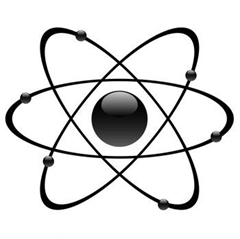It would be interesting to know what article and what the author means by definitive.

This lesson can be used as an introduction to radioactivity. Students should have familiarity with the scientific notation and the units milli, micro, and nano. Students will be introduced to being science/math detectives by trying to figure out the relationship of organisms using graphs. Students then are introduced to the controversy around the Shroud of Turin, which has been carbon dated. What is the process of carbon dating, and can the results be believed? Through the use of an interactive Web site, students will learn about C-14 and C-14 dating. Then students will analyze an article written about the C-14 dating of the Shroud of Turin and draw conclusions. Although the results are definitive, they can be used to support either point of view.

Radioaktivitas bagian 2 – Bahan Ajar Fisika Berbasis TIK